# Engineering Mechanics - Structural Analysis

### Exercise :: Structural Analysis - General Questions

• Structural Analysis - General Questions
11.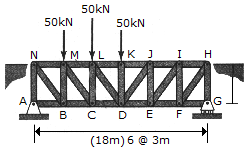The Pratt bridge truss is subjected to the loading shown. Determine the force in members CD, CL and ML, and indicate whether these members are in tension or compression.

 A. CL = 100 kN T, ML = 150 kN T, CD = 150 kN C B. CL = 50 kN C, ML = 112.5 kN C, CD = 112.5 kN T C. CL = 100 kN C, ML = 150 kN C, CD = 150 kN T D. CL = 50 kN T, ML = 112.5 kN T, CD = 112.5 kN C

Explanation:

No answer description available for this question. Let us discuss.

12.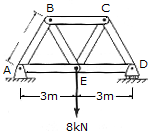Compute the force in each member of the Warren truss and indicate whether the members are in tension or compression. All the members are 3 m long.

 A. AB = CD = 8.00 kN C, AE = DE = 6.92 kN T, BE = CE = 8.00 kN T, BC = 6.92 kN C B. AB = CD = 4.62 kN C, AE = DE = 2.31 kN T, BE = CE = 4.62 kN T, BC = 2.31 kN C C. AB = CD = 8.00 kN T, AE = DE = 6.92 kN C, BE = CE = 8.00 kN C, BC = 6.92 kN T D. AB = CD = 4.62 kN T, AE = DE = 2.31 kN C, BE = CE = 4.62 kN C, BC = 2.31 kN T

Explanation:

No answer description available for this question. Let us discuss.

13.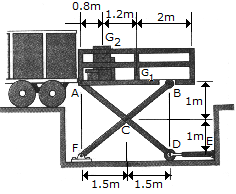The scissors lift consists of two sets of symmetrically placed cross members (one in front that is shown and one behind that is not shown) and two hydraulic cylinders (front [labeled DE] and back [not shown]). The uniform platform has a mass of 60 kg with a center of gravity at G1. The 85 kg load (center of gravity at G2) is centered front to back. Determine the force in each of the hydraulic cylinders necessary to maintain equilibrium. These are rollers at B and D.

 A. DE = 1.067 kN C B. DE = 1.139 kN C C. DE = 0.606 kN C D. DE = 1.207 kN C

Explanation:

No answer description available for this question. Let us discuss.

14.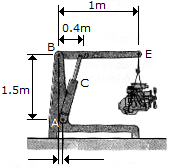The jack shown supports a 350-kg automobile engine. Determine the compression in the hydraulic cylinder C and the magnitude of force that pin B exerts on the horizontal member BDE.

 A. FC = 8.75 kN, FB = 5.43 kN B. FC = 5.15 kN, FB = 5.15 kN C. FC = 8.58 kN, FB = 5.15 kN D. FC = 5.25 kN, FB = 7.36 kN

Explanation:

No answer description available for this question. Let us discuss.

15.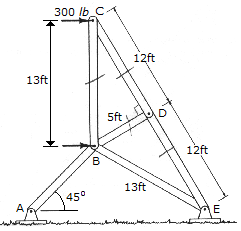A sign is subjected to a wind loading that exerts horizontal forces of 300 lb on joints B and C of one of the side supporting trusses. Determine the force in members BC, CD, DB, and DE of the truss and state whether the members are in tension or compression.

 A. CB = 720 lb C, CD = 780 lb T, DB = 0, DE = 780 lb T B. CB = 720 lb T, CD = 780 lb C, DB = 0, DE = 780 lb C C. CB = 125 lb T, CD = 325 lb C, DB = 0, DE = 325 lb C D. CB = 125 lb C, CD = 325 lb T, DB = 0, DE = 325 lb T